Home Automation

design a smart lock for your houseThings used in this project

Hardware componentsAndroid device
×1Raspberry Pi 3 Model B
×1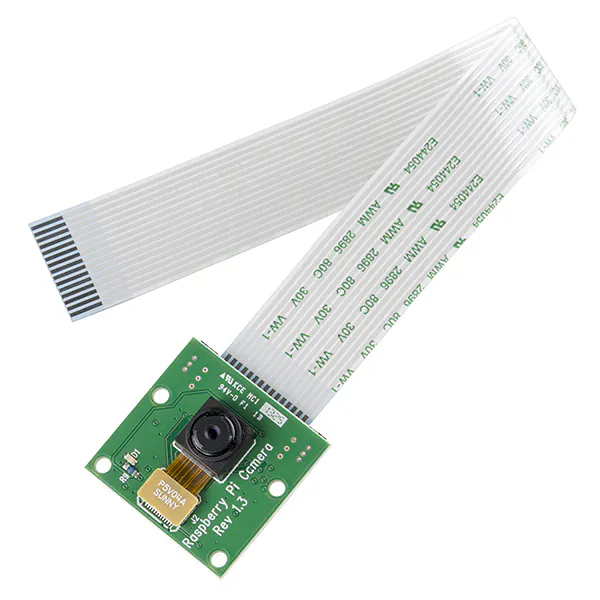Raspberry Pi Camera Module
×1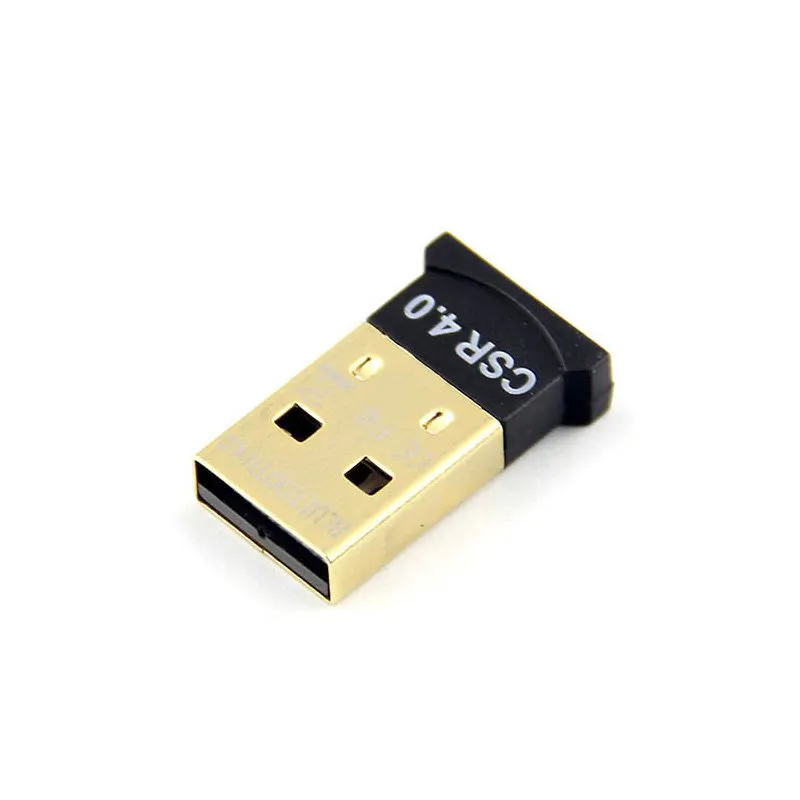mbientLab Bluetooth to USB Dongle
×1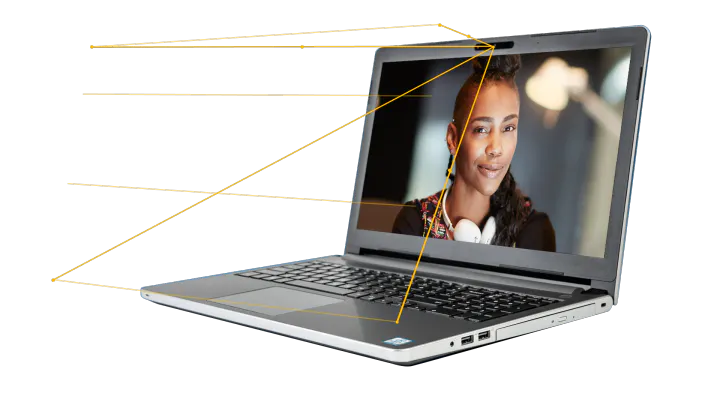Intel RealSense Camera
×1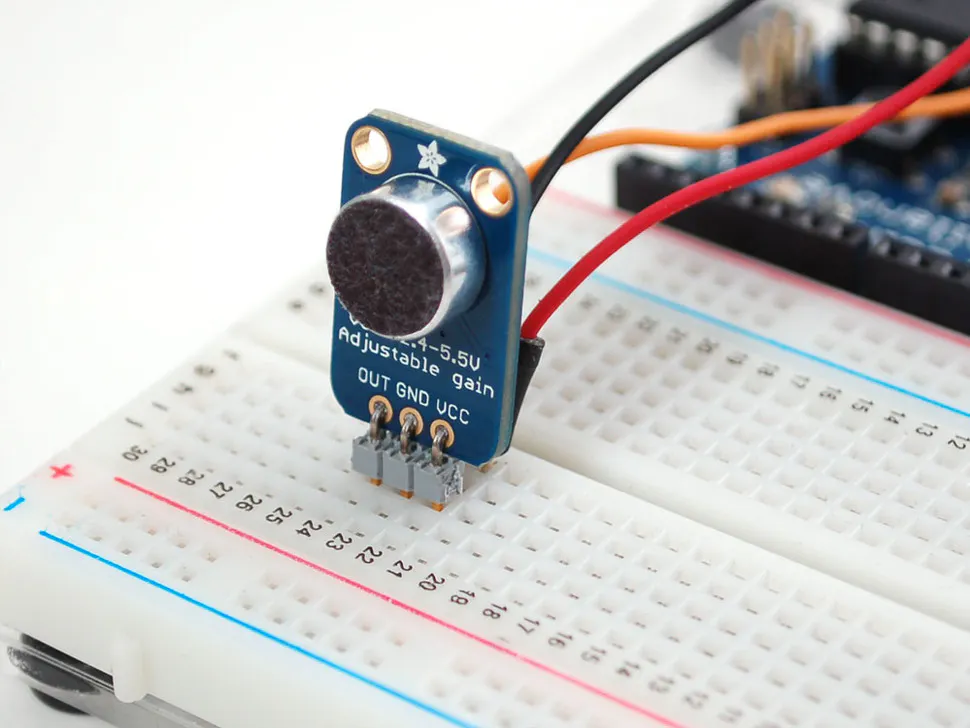×1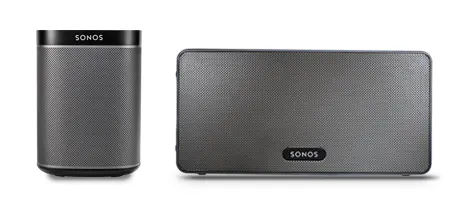Sonos speakers
×1Amazon Web Services AWS CloudWatch
×1

Software apps and online servicesCode

get face from camera

Python
import cv2
import os
import sys
import random

out_dir = './my_faces'
if not os.path.exists(out_dir):
os.makedirs(out_dir)

def relight(img, alpha=1, bias=0):
w = img.shape
h = img.shape
#image = []
for i in range(0,w):
for j in range(0,h):
for c in range(3):
tmp = int(img[j,i,c]*alpha + bias)
if tmp > 255:
tmp = 255
elif tmp < 0:
tmp = 0
img[j,i,c] = tmp
return img

camera = cv2.VideoCapture(0)

n = 1
while 1:
if (n <= 10000):
print('It`s processing %s image.' % n)

gray_img = cv2.cvtColor(img, cv2.COLOR_BGR2GRAY)
faces = haar.detectMultiScale(gray_img, 1.3, 5)
for f_x, f_y, f_w, f_h in faces:
face = img[f_y:f_y+f_h, f_x:f_x+f_w]
face = cv2.resize(face, (64,64))
'''
if n % 3 == 1:
face = relight(face, 1, 50)
elif n % 3 == 2:
face = relight(face, 0.5, 0)
'''
face = relight(face, random.uniform(0.5, 1.5), random.randint(-50, 50))
cv2.imshow('img', face)
cv2.imwrite(out_dir+'/'+str(n)+'.jpg', face)
n+=1
key = cv2.waitKey(30) & 0xff
if key == 27:
break
else:
break

Credits

aliceding

1 project • 1 follower